# Power Supplies

Next Page: Transducers

Also See: AC/DC | Diodes | Capacitors

## Types of Power Supply

There are many types of power supply. Most are designed to convert high voltage AC mains electricity to a suitable low voltage supply for electronics circuits and other devices. A power supply can by broken down into a series of blocks, each of which performs a particular function.

For example a 5V regulated supply: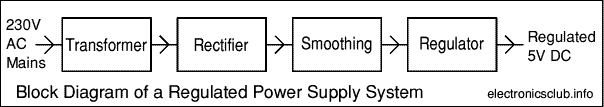• Transformer - steps down high voltage AC mains to low voltage AC.
• Rectifier - converts AC to DC, but the DC output is varying.
• Smoothing - smooths the DC from varying greatly to a small ripple.
• Regulator - eliminates ripple by setting DC output to a fixed voltage.

Power supplies made from these blocks are described below with a circuit diagram and a graph of their output:

### Transformer only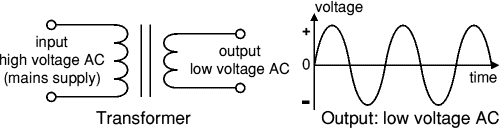The low voltage AC output is suitable for lamps, heaters and special AC motors. It is not suitable for electronic circuits unless they include a rectifier and a smoothing capacitor.

See: Transformer

### Transformer + Rectifier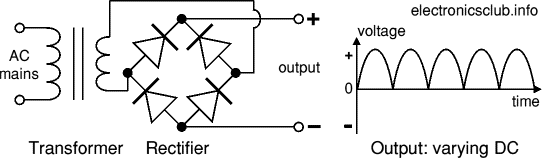The varying DC output is suitable for lamps, heaters and standard motors. It is not suitable for electronic circuits unless they include a smoothing capacitor.

See: Transformer | Rectifier

### Transformer + Rectifier + Smoothing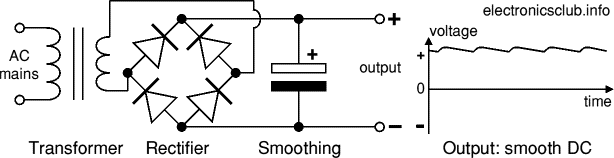The smooth DC output has a small ripple. It is suitable for most electronic circuits.

### Transformer + Rectifier + Smoothing + Regulator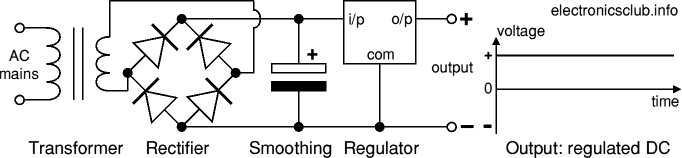The regulated DC output is very smooth with no ripple. It is suitable for all electronic circuits.

## Transformer

Transformers convert AC electricity from one voltage to another with little loss of power. Transformers work only with AC and this is one of the reasons why mains electricity is AC.

Step-up transformers increase voltage, step-down transformers reduce voltage. Most power supplies use a step-down transformer to reduce the dangerously high mains voltage (230V in UK) to a safer low voltage.

Transformers waste very little power so the power out is (almost) equal to the power in. Note that as voltage is stepped down current is stepped up.

The input coil is called the primary and the output coil is called the secondary. There is no electrical connection between the two coils, instead they are linked by an alternating magnetic field created in the soft-iron core of the transformer. The two lines in the middle of the circuit symbol represent the core.

Rapid Electronics: Transformers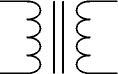Transformer circuit symbol

### Turns ratio

The ratio of the number of turns on each coil, called the turns ratio, determines the ratio of the voltages. A step-down transformer has a large number of turns on its primary (input) coil which is connected to the high voltage mains supply, and a small number of turns on its secondary (output) coil to give a low output voltage.

 turns ratio = Vp = Np Vs Ns
 power out = power in Vs × Is = Vp × Ip

Vp = primary (input) voltage
Np = number of turns on primary coil
Ip  = primary (input) current

Vs = secondary (output) voltage
Ns = number of turns on secondary coil
Is  = secondary (output) current

## Rectifier

There are several ways of connecting diodes to make a rectifier to convert AC to DC. The bridge rectifier is the most important and it produces full-wave varying DC. A full-wave rectifier can also be made from just two diodes if a centre-tap transformer is used, but this method is rarely used now that diodes are cheaper. A single diode can be used as a rectifier but it only uses the positive (+) parts of the AC wave to produce half-wave varying DC.

### Bridge rectifier

A bridge rectifier can be made using four individual diodes, but it is also available in packages containing the four diodes required. It is called a full-wave rectifier because it uses all the AC wave (both positive and negative sections). Alternate pairs of diodes conduct, this changes over the connections so the alternating directions of AC are converted to the one direction of DC.

1.4V is used up in a bridge rectifier because there is 0.7V across each diode when conducting and there are always two diodes conducting, as shown in the diagram.

Bridge rectifiers are rated by the maximum current they can pass and the maximum reverse voltage they can withstand. Their voltage rating must be at least three times the RMS voltage of the supply so the rectifier can withstand the peak voltages. Please see the Diodes page for more details, including pictures of bridge rectifiers.

Rapid Electronics: Bridge rectifiers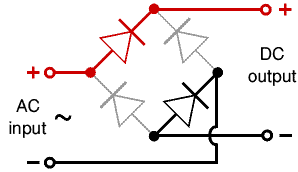Bridge rectifier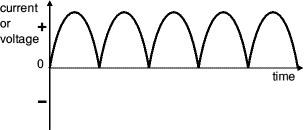Output: full-wave varying DC
(using all the AC wave)

### Single diode rectifier

A single diode can be used as a rectifier but this produces half-wave varying DC which has gaps when the AC is negative. It is hard to smooth this sufficiently well to supply electronic circuits unless they require a very small current so the smoothing capacitor does not significantly discharge during the gaps. Please see the Diodes page for some examples of rectifier diodes.

Rapid Electronics: Rectifier diodes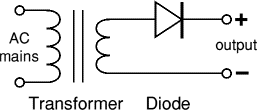Single diode rectifier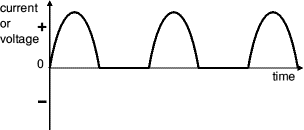Output: half-wave varying DC
(using only half the AC wave)

## Smoothing

Smoothing is performed by a large value electrolytic capacitor connected across the DC supply to act as a reservoir, supplying current to the output when the varying DC voltage from the rectifier is falling. The diagram shows the unsmoothed varying DC (dotted line) and the smoothed DC (solid line). The capacitor charges quickly near the peak of the varying DC, and then discharges as it supplies current to the output.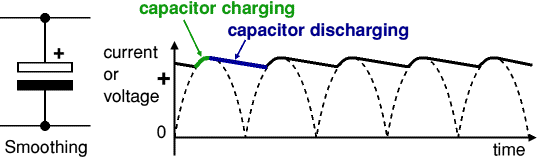Note that smoothing significantly increases the average DC voltage to almost the peak value (1.4 × RMS value). For example 6V RMS AC is rectified to full wave DC of about 4.6V RMS (1.4V is lost in the bridge rectifier), with smoothing this increases to almost the peak value giving 1.4 × 4.6 = 6.4V smooth DC.

Smoothing is not perfect due to the capacitor voltage falling a little as it discharges, giving a small ripple voltage. For many circuits a ripple which is 10% of the supply voltage is satisfactory and the equation below gives the required value for the smoothing capacitor. A larger capacitor will give less ripple. The capacitor value must be doubled when smoothing half-wave DC.

Rapid Electronics: Electrolytic Capacitors

Smoothing capacitor, C, for 10% ripple:

 C = 5 × Io Vs × f

where:
C  = smoothing capacitance in farads (F)
Io  = output current in amps (A)
Vs = supply voltage in volts (V), this is the peak value of the unsmoothed DC
f    = frequency of the AC supply in hertz (Hz), this is 50Hz in the UK

## Regulator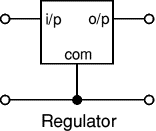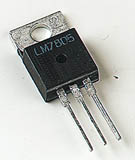Voltage regulator ICs are available with fixed (typically 5, 12 and 15V) or variable output voltages. They are also rated by the maximum current they can pass. Negative voltage regulators are available, mainly for use in dual supplies. Most regulators include some automatic protection from excessive current ('overload protection') and overheating ('thermal protection').

Many of the fixed voltage regulator ICs have 3 leads and look like power transistors, such as the 7805 +5V 1A regulator shown on the right. They include a hole for attaching a heatsink if necessary.

Rapid Electronics: 7805 regulator

Voltage regulator photograph © Rapid Electronics

### Zener diode regulator

For low current power supplies a simple voltage regulator can be made with a resistor and a zener diode connected in reverse as shown in the diagram. Zener diodes are rated by their breakdown voltage Vz and maximum power Pz (typically 400mW or 1.3W).

The resistor limits the current (like an LED resistor). The current through the resistor is constant, so when there is no output current all the current flows through the zener diode and its power rating Pz must be large enough to withstand this.

Rapid Electronics: Zener Diodes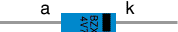zener diode
a = anode, k = cathode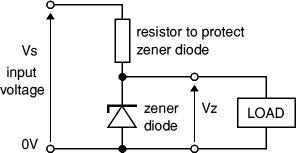### Choosing a zener diode and resistor

These are the steps for choosing a zener diode and resistor:

1. The zener voltage Vz is the output voltage required
2. The input voltage Vs must be a few volts greater than Vz
(this is to allow for small fluctuations in Vs due to ripple)
3. The maximum current Imax is the output current required plus 10%
4. The zener power Pz is determined by the maximum current:  Pz > Vz × Imax
5. The resistor resistance:  R = (Vs - Vz) / Imax
6. The resistor power rating:  P > (Vs - Vz) × Imax

The example shows how to use these steps to choose a zener diode and resistor with suitable values and power ratings.

#### For example

If the required output voltage is 5V and output current is 60mA:

1. Vz = 4.7V (the nearest value available)
2. Vs = 8V (a few volts more than Vz)
3. Imax = 66mA (current plus 10%)
4. Pz > 4.7V × 66mA = 310mW, choose Pz = 400mW
5. R = (8V - 4.7V) / 66mA = 0.05k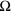= 50,
choose R = 476. Resistor power rating P > (8V - 4.7V) × 66mA = 218mW, choose P = 0.5W

## Dual Supplies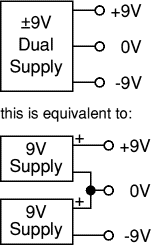Some electronic circuits require a power supply with positive and negative outputs as well as zero volts (0V). This is called a 'dual supply' because it is like two ordinary supplies connected together as shown in the diagram.

Dual supplies have three outputs, for example a ±9V supply has +9V, 0V and -9V outputs.

Rapid Electronics: Power Supplies

Next Page: Transducers | Study